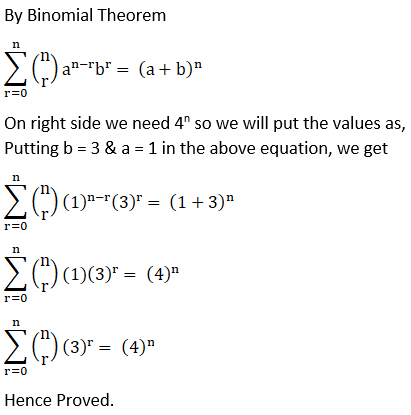# NCERT Solutions For Class 11 Math Chapter – 8 Exercise – 8.1

NCERT Solutions For Class 11 Math Chapter – 8 Exercise – 8.1

Expand each of the expressions in Exercises 1 to 5.

1. (1 – 2x)5

Solution:

From binomial theorem expansion we can write as

(1 – 2x)5

5C(1)5 – 5C(1)4 (2x) + 5C(1)(2x)2 – 5C(1)(2x)3 + 5C(1)1 (2x)4 – 5C(2x)5

= 1 – 5 (2x) + 10 (4x)2 – 10 (8x3) + 5 ( 16 x4) – (32 x5)

= 1 – 10x + 40x2 – 80x3 + 80x4– 32x5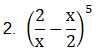Solution:

From binomial theorem, given equation can be expanded as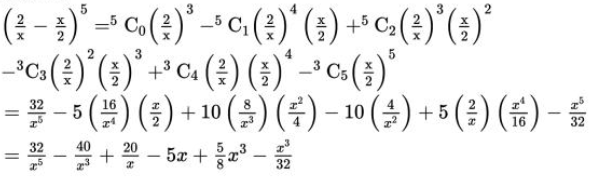3.

(2x – 3)6

Solution:

From binomial theorem, given equation can be expanded as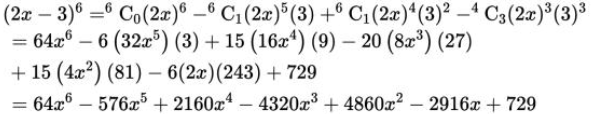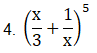Solution:

From binomial theorem, given equation can be expanded as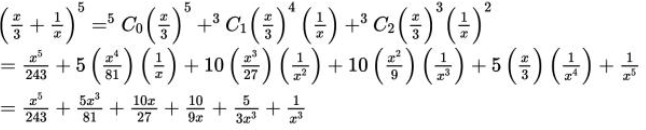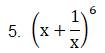Solution:

From binomial theorem, given equation can be expanded as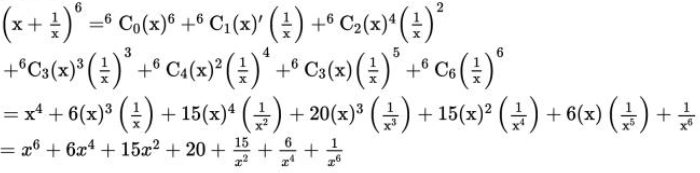6. (96)3

Solution:

Given (96)3

96 can be expressed as the sum or difference of two numbers and then binomial theorem can be applied.

The given question can be written as 96 = 100 – 4

(96)3 = (100 – 4)3

3C0 (100)3 – 3C1 (100)2 (4) – 3C2 (100) (4)2– 3C3 (4)3

= (100)3 – 3 (100)2 (4) + 3 (100) (4)2 – (4)3

= 1000000 – 120000 + 4800 – 64

= 884736

7. (102)5

Solution:

Given (102)5

102 can be expressed as the sum or difference of two numbers and then binomial theorem can be applied.

The given question can be written as 102 = 100 + 2

(102)5 = (100 + 2)5

5C0 (100)5 + 5C1 (100)4 (2) + 5C2 (100)3 (2)2 + 5C3 (100)2 (2)3 + 5C4 (100) (2)4 + 5C5 (2)5

= (100)5 + 5 (100)4 (2) + 10 (100)3 (2)2 + 5 (100) (2)3 + 5 (100) (2)4 + (2)5

= 1000000000 + 1000000000 + 40000000 + 80000 + 8000 + 32

= 11040808032

8. (101)4

Solution:

Given (101)4

101 can be expressed as the sum or difference of two numbers and then binomial theorem can be applied.

The given question can be written as 101 = 100 + 1

(101)4 = (100 + 1)4

4C0 (100)4 + 4C1 (100)3 (1) + 4C2 (100)2 (1)2 + 4C3 (100) (1)2 + 4C(1)4

= (100)4 + 4 (100)3 + 6 (100)2 + 4 (100) + (1)4

= 100000000 + 400000 + 60000 + 400 + 1

= 1040604001

9. (99)5

Solution:

Given (99)5

99 can be written as the sum or difference of two numbers then binomial theorem can be applied.

The given question can be written as 99 = 100 -1

(99)5 = (100 – 1)5

5C0 (100)5 – 5C1 (100)4 (1) + 5C2 (100)3 (1)2 – 5C3 (100)2 (1)3 + 5C4 (100) (1)4 – 5C5 (1)5

= (100)5 – 5 (100)4 + 10 (100)3 – 10 (100)2 + 5 (100) – 1

= 1000000000 – 5000000000 + 10000000 – 100000 + 500 – 1

= 9509900499

10. Using Binomial Theorem, indicate which number is larger (1.1)10000 or 1000.

Solution:

By splitting the given 1.1 and then applying binomial theorem, the first few terms of (1.1)10000 can be obtained as

(1.1)10000 = (1 + 0.1)10000

= (1 + 0.1)10000 C(1.1) + other positive terms

= 1 + 10000 × 1.1 + other positive terms

= 1 + 11000 + other positive terms

> 1000

(1.1)10000 > 1000

11. Find (a + b)4 – (a – b)4. Hence, evaluate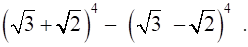Solution:

Using binomial theorem the expression (a + b)4 and (a – b)4, can be expanded

(a + b)4 = 4C0 a4 + 4C1 a3 b + 4C2 a2 b2 + 4C3 a b3 + 4C4 b4

(a – b)4C0 a4 – 4C1 a3 b + 4C2 a2 b2 – 4C3 a b3 + 4C4 b4

Now (a + b)4 – (a – b)4 = 4C0 a4 + 4C1 a3 b + 4C2 a2 b2 + 4C3 a b3 + 4C4 b4 – [4C0 a4 – 4C1 a3 b + 4C2 a2 b2 – 4C3 a b3 + 4C4 b4]

= 2 (4C1 a3 b + 4C3 a b3)

= 2 (4a3 b + 4ab3)

= 8ab (a2 + b2)

Now by substituting a = √3 and b = √2 we get

(√3 + √2)4 – (√3 – √2)4 = 8 (√3) (√2) {(√3)2 + (√2)2}

= 8 (√6) (3 + 2)

= 40 √6

12. Find (x + 1)6 + (x – 1)6. Hence or otherwise evaluate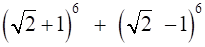Solution:

Using binomial theorem the expressions, (x + 1)6 and (x – 1)6 can be expressed as

(x + 1)6 = 6C0 x6 + 6C1 x5 + 6C2 x4 + 6C3 x3 + 6C4 x2 + 6C5 x + 6C6

(x – 1)6 = 6C0 x6 – 6C1 x5 + 6C2 x4 – 6C3 x3 + 6C4 x2 – 6C5 x + 6C6

Now, (x + 1)6 – (x – 1)6 = 6C0 x6 + 6C1 x5 + 6C2 x4 + 6C3 x3 + 6C4 x2 + 6C5 x + 6C6 – [6C0 x6 – 6C1 x5 + 6C2 x4 – 6C3 x3 + 6C4 x2 – 6C5 x + 6C6]

= 2 [6C0 x6C2 x4 + 6C4 x2 + 6C6]

= 2 [x6 + 15x4 + 15x2 + 1]

Now by substituting x = √2 we get

(√2 + 1)6 – (√2 – 1)6 = 2 [(√2)6 + 15(√2)4 + 15(√2)2 + 1]

= 2 (8 + 15 × 4 + 15 × 2 + 1)

= 2 (8 + 60 + 30 + 1)

= 2 (99)

= 19813. Show that 9n+1 – 8n – 9 is divisible by 64, whenever n is a positive integer.

Solution:

In order to show that 9n+1 – 8n – 9 is divisible by 64, it has to be show that 9n+1 – 8n – 9 = 64 k, where k is some natural number

Using binomial theorem,

(1 + a)m = mC0 + mC1 a + mC2 a2 + …. + m am

For a = 8 and m = n + 1 we get

(1 + 8)n+1 = n+1C0 + n+1C1 (8) + n+1C2 (8)2 + …. + n+1 n+1 (8)n+1

9n+1 = 1 + (n + 1) 8 + 82 [n+1C2 + n+1C3 (8) + …. + n+1 n+1 (8)n-1]

9n+1 = 9 + 8n + 64 [n+1C2 + n+1C3 (8) + …. + n+1 n+1 (8)n-1]

9n+1 – 8n – 9 = 64 k

Where k = [n+1C2 + n+1C3 (8) + …. + n+1 n+1 (8)n-1] is a natural number

Thus, 9n+1 – 8n – 9 is divisible by 64, whenever n is positive integer.

Hence the proof

14. Prove that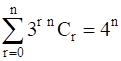Solution: